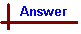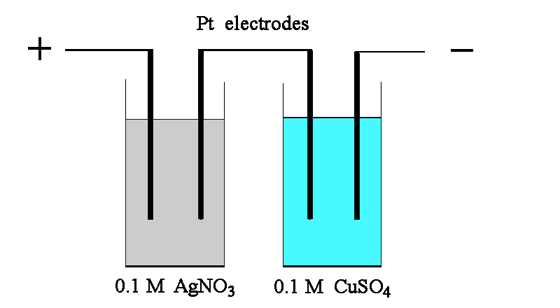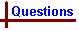Electrolysis Your feedback on these self-help problems is appreciated. Click here to send an e-mail. Shortcut to Questions Q: 1 2 3 4 5 6 7 8 1 How many (a) faradays, (b) coulombs are required to deposit 0.587 g of Ni from a solution of Ni2+ ?2 From a dilute solution of potassium tricyanocuprate(I), K2[Cu(CN)3], a uniform current deposits 0.586 g of copper in 108 minutes. What volume of hydrogen measured over water at 296 K and 746.0 mmHg would the same current liberate from dilute sulfuric acid in 81.0 minutes? (The equilibrium vapour pressure of water at 296 K = 20.9 mmHg.)3 In the electrolysis of 0.100 M copper(II) sulfate solution, copper is deposited. How long would it be necessary to pass a 0.100 ampere current in order to deposit all of the copper from 1.00 litre of this solution?4 Three electrolysis cells are connected in series. They contain, respectively, solutions of copper(II) nitrate, silver nitrate, and chromium(III) sulfate. If 1.00 g of copper is electrochemically deposited in the first cell, calculate the mass of silver and chromium deposited in the other cells.5 A constant current of 3.7 milliampere is passed through molten sodium chloride for 9.0 minutes. The sodium produced is allowed to react with water (500 mL). What is the pH of the resulting solution?6 A constant current of 0.126 ampere was passed through a dilute sulfuric acid solution for 149 minutes. The hydrogen produced was collected over mercury and the pressure adjusted to that of the surroundings (751 mmHg). The room temperature was 291 K. What was the volume of hydrogen produced?7 One of the experimental ways of determining the Avogadro constant is by electrolysis. A current of 1.00 ampere is passed for 1.00 hour through a solution of potassium iodide. The weight of iodine liberated at the anode is 4.7135 g and there is no other anodic product. If the charge on the electron is 1.602 x 10-19 coulomb, find from this set of data the Avogadro constant, NA, correct to three significant figures. The atomic weight of iodine is 126.90.8Two electrolytic cells each containing two platinum electrodes were connected in series and a current of 0.500 ampere was passed for 16 minutes and 5 seconds. Cell 1 contained 0.100 M AgNO3 and cell 2 contained 0.100 M CuSO4. Calculate the following: (a) The change in mass of the platinum cathode in cell 1. (b) The volume of gas at 1.00 atmosphere and 273 K liberated at the platinum anode in cell 1. (c) The volume of gas at 1.00 atmosphere and 273 K liberated at the platinum anode in cell 2. (d) The change in mass of the platinum cathode in cell 2.Electrolysis (Answers) 1 The reaction equation is Ni2+ + 2e- → Ni From the stoichiometry of this equation, one mole of Ni produced requires the passage of two moles of electrons in the electrolysis. Mass of nickel produced = 0.587 g ∴ moles of Ni = mass/atomic wt = 0.587/58.71 = 1.00 x 10-2 mol and moles of electrons required = 2 x 1.00 x 10-2 = 2.00 x 10-2 mol. One faraday of electrical charge (F) is one mole of electrons (i.e. 6.02 x 1023 electrons - note that only the Avogadro number definition can apply to moles of electrons). ∴ number of faradays required = 2.00 x 10-2 F One coulomb of charge (C) is the total charge involved when a current of one amp flows for one second. i.e. 1 amp = 1 coulomb flowing for 1 second. By experiment, one faraday is found to contain 96487 C per mole of electrons, the Faraday constant, F. ∴ coulombs in 2.00 x 10-2 faradays = 96487 x 2.00 x 10-2 = 1.93 x 103 C2 The first task in this question is to calculate from the amount of copper released, the current used in the electrolysis reactions. Then in the second part, knowing the current and the time it flows, the moles of electrons supplied to the H+ ions can be deduced. Then the moles of H2 released and its volume under the stated conditions can be obtained. From the first electrolysis, deduce the moles of electrons: The reaction equation is Cu+ + e- → Cu from which, one mole of electrons releases one mole of Cu. Mass of copper = 0.586 g ∴ moles of Cu = 0.586 / 63.55 = 9.221 x 10-3 mol and moles of electrons also = 9.221 x 10-3 mol. Convert moles of electrons to coulombs: Q = F x moles of electrons = 96487 x 9.221 x 10-3 C = 8.897 x 102 C From the coulombs of charge, calculate the current: By the definition of the coulomb (see Q 1 answer), charge in coulombs = current in amps x time in seconds or Q = I x t ∴ I = Q / t = 8.897 x 102 / (108 x 60.0) =0.1373 amps Note that time must be expressed in seconds as required by the definition of the coulomb. Calculate the moles of electrons this current provides in 81.0 minutes: From Q = I x t, in 81.0 minutes (= 81.0 x 60.0 s), Q = 0.1373 x 81.0 x 60.0 = 6.673 x 102 C. Converting coulombs to moles of electrons, moles of electrons = coulombs / F = 6.673 x 102 / 96487 = 6.916 x 10-3 mol. Calculate the moles of H2 released: The reaction equation is 2H+ + 2e- → H2 from which, one mole of H2 formed requires two moles of electrons. ∴ moles of H2 = ½ x 6.916 x 10-3 = 3.458 x 10-3 mol Calculate the volume of hydrogen gas formed: From the ideal gas equation PV = nRT, ∴ V = nRT / P n = 3.458 x 10-3 mol R = 8.314 J K-1 mol-1 T = 296 K P = (746.0 - 20.9) mmHg = 725.1 mmHg or (725.1 / 760.0) atm or (725.1 / 760.0) x 101.3 kPa = 96.65 kPa. (Note that the total pressure must be reduced by the equilibrium vapour pressure of the water over which the hydrogen is collected in order to obtain the true pressure of hydrogen in the container.) Substituting this data, V = (3.458 x 10-3 x 8.314 x 296) / 96.65 = 8.80 x 10-2 L3 The reaction equation is Cu2+ + 2e- → Cu From the stoichiometry of this equation, one mole of Cu2+ requires the passage of two moles of electrons in the electrolysis. Moles of Cu2+ in 1.00 L of 0.100 M solution = 0.100 mol. ∴ moles of electrons required = 0.200 mol or 0.200 F of charge. Q = 96487 x F = 96487 x 0.200 coulombs = 19297 C. Q = I x t for Q in coulombs, I in amps and t in seconds I = 0.100 amps ∴ t = Q / I = 19297 / 0.100 = 1.93 x 105 seconds or 1.93 x 105 / (60.0 x 60.0) hours = 53.6 hours.4 The reaction equations are Cu2+ + 2e- → Cu Ag+ + e- → Ag Cr3+ + 3e- → Cr From the stoichiometry of these equations, one mole of electrons would deposit ½ mole of Cu in the electrolysis. As the cells are in series, the same current flows through all three cells and one mole of electrons would produce one mole of Ag and 1/3 mole of Cr from their solutions respectively. The moles of electrons used = 2 x moles of Cu deposited. Moles of Cu deposited = 1.00 / 63.55 = 1.574 x 10-2 mol, so moles of electrons passed = 2 x 1.574 x 10-2 = 3.148 x 10-2 mol. In the silver cell: Moles of Ag deposited = moles of electrons passed = 3.148 x 10-2 mol Mass of silver deposited = 3.148 x 10-2 x 107.87 g = 3.40 g In the chromium cell: Moles of Cr deposited = (1/3) x moles of electrons passed = (3.148 x 10-2) / 3 = 1.049 x 10-2 mol Mass of chromium deposited = 1.049 x 10-2 x 52.00 g = 0.546 g5 The reaction equation is Na+ + e- → Na From the stoichiometry of this equation, one mole of Na deposited requires the passage of one mole of electrons in the electrolysis. The sodium deposited is then reacted with water according to the equation Na + H2O → Na+ + OH- + ½H2 From this equation, one mole of OH- ions in the solution is formed from one mole of Na which in turn was deposited by the passage of one mole of electrons. ∴ moles of OH- = moles of electrons passed Moles of electrons passed = Q / F = I x t / F I = 3.7 ma = 3.7 x 10-3 amps (note that the units of current must be amps) t = 9.0 minutes = 9.0 x 60.0 seconds ∴ moles of electrons = (3.7 x 10-3 x 9.0 x 60.0) / 96487 mol = 2.07 x 10-5 mol. The solution contains 2.07 x 10-5 mol of OH- ions in 500 mL of water. ∴ [OH-] = (2.07 x 10-5) / 0.500 M = 4.14 x 10-5 M pOH = -log[OH-] = 4.38 pH = 14 00 - pOH = 9.626 The reaction equation is 2H+ + 2e- → H2 From the stoichiometry of this equation, one mole of H2 produced requires the passage of two moles of electrons in the electrolysis. Calculate the moles of electrons passed. Moles of electrons = I x t / F I = 0.126 amps t = 149 x 60 seconds = 8940 seconds ∴ moles of electrons = 0.126 x 8940 / 96487 = 1.167 x 10-2 mol. Calculate the moles of H2 produced. From the equation above, moles of H2 = ½ x moles of electrons = (1.167 x 10-2) / 2 = 5.84 x 10-3 mol Calculate the volume of hydrogen produced. Use the ideal gas equation rewritten as V = nRT / P where n = 5.84 x 10-3 mol R = 8.314 JK-1mol-1 T = 291 K P = 751 mmHg = 751 / 760 atm = (751 / 760) x 101.3 kPa = 100.1 kPa [Note that there is no correction needed for the vapour pressure of mercury as it is negligible] ∴ V = (5.84 x 10-3 x 8.314 x 291) / 100.1 = 0.141 L7 The reaction equation is 2I- → I2 + 2e- From the stoichiometry of this equation, one mole of I2 produced requires the passage of two moles of electrons in the electrolysis. Calculate the moles of I2 formed and thus moles of electrons passed. Moles of I2 = mass of I2 / GFW of I2 = 4.7135 / (2 x 126.90) = 1.8572 x 10-2 mol ∴ moles of electrons = 2 x 1.8572 x 10-2 = 3.7143 x 10-2 mol Calculate the total charge passed and thus the number of electrons. Q = I x t I = 1.00 amp t = 1.00 hour = 1.00 x 60.00 x 60.00 = 3600 sec (note the units of time must be seconds) ∴ Q = 1.00 x 3600 = 3600 C Given that the charge on one electron = 1.602 x 10-19 C, ∴ number of electrons passed = 3600 / (1.602 x 10-19) = 2.247 x 1022 electrons Thus deduce the number of electrons in one mole of electrons. As 2.247 x 1022 electrons = 3.7143 x 10-2 mol, then 1.00 mol = (2.247 x 1022) / (3.7143 x 10-2) electrons = 6.05 x 1023 electrons. ∴ NA = 6.05 x 1023.8 Terminology: Cathode is where reduction occurs and is ∴ the negative electrode Anode is where oxidation occurs and is ∴ the positive electrode. Cell diagramSet out the cell reactions for both cells. The reduction potentials for both Ag+ and Cu2+ are larger than that for H+, so Ag and Cu will be deposited at the cathodes before H+ is reduced to H2. The cell reactions are as follows. Cell 1 cathode: 2Ag+ + 2e- → 2Ag Cell 1 anode: H2O → 2H+ + ½O2(g) + 2e- Cell 2 cathode: Cu2+ + 2e- → Cu Cell 2 anode: H2O → 2H+ + ½O2(g) + 2e- Calculate the moles of electrons passed. Moles of electrons = I x t / F I = 0.500 amp t = 16 min 5 sec = (16 x 60) + 5 sec = 965 sec ∴ moles of electrons = (0.500 x 965) / 96487 = 5.00 x 10-3 mole Using the stoichiometry set out above, deduce the quantities of products. (a) Cell 1 cathode: In cell 1, moles of Ag formed = moles of electrons passed Mass of silver = moles x atomic wt = 5.00 x 10-3 x 107.87 g = 0.539 g (increase in mass of electrode). (b) Cell 1 anode: In cell 1, moles of O2 released = ¼ x moles of electrons passed = (5.00 x 10-3) / 4 mole = 1.25 x 10-3 mol Volume of oxygen released = nRT / P T = 273 K P = 1.00 atm = 101.3 kPa R = 8.314 ∴ V = (1.25 x 10-3 x 8.314 x 273) / 101.3 = 0.0280 L or 28.0 mL (c) Cell 2 anode: In cell 2, the same volume of oxygen is liberated at the anode as the same current passes through both cells. (d) Cell 2 cathode: In cell 2, moles of Cu formed = ½ x moles of electrons passed = 2.50 x 10-3 mol. Mass of copper = moles x atomic wt = 2.50 x 10-3 x 63.55 g = 0.159 g (increase in mass of electrode).